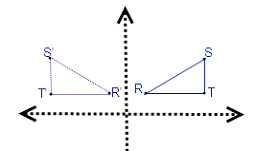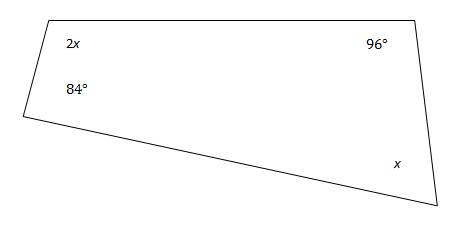# ACT Math Practice Test 1

Start your math test prep now with our free ACT Math Practice Tests. Topics covered include pre-algebra, elementary algebra, intermediate algebra, coordinate geometry, plane geometry, and trigonometry.

Difficulty Level – 1: Easy

Directions: Solve each problem and then click on the correct answer. You are permitted to use a calculator on this test.

Congratulations - you have completed . You scored %%SCORE%% out of %%TOTAL%%. Your performance has been rated as %%RATING%%
 Question 1
If 4x + 3x − 2(x + 5) = −9, then x = ?

 A −1/2 B 0 C 1/5 D 2/3 E 4/5
Question 1 Explanation:
The correct answer is (C). Evaluate the expression by first distributing the −2 through the parentheses:
7x − 2x − 10 = −9
5x = 1
x = 1/5
 Question 2
If the ratio of milk cartons to juice boxes is 13:x and there are 39 milk cartons and 18 juice boxes, what is the value of x?

 A 4 B 6 C 8 D 10 E 12
Question 2 Explanation:
The correct answer is (B). Solve this problem by setting up a proportion. We are told the ratio of milk to juice is 13:x and that there are 39 milk and 18 juice so → 13/x = 39/18, cross multiply and solve for x to get 6.
 Question 3
A triangle, RST, is reflected across the y-axis to form the triangle R'S'T' in the standard (x,y) coordinate plane; thus, R reflects to R'. The coordinates of point T are (j,k). What are the coordinates of point T'?

 A (−j, k) B (j, −k) C (−j, −k) D (k, j) E It cannot be determined.
Question 3 Explanation:
The correct answer is (A). When you reflect a point across the y-axis, the value of its x-value is made negative. So: (j,k) → (−j,k)

To visualize draw a picture:Question 4
How many of the numbers between 20 and 40 are prime numbers?

 A 3 B 4 C 5 D 6 E 7
Question 4 Explanation:
The correct answer is (B). A prime number is a number with no factors other than 1 and itself. Therefore, we can solve this problem by listing the numbers from 20 to 40 and eliminating those which are clearly divisible by some other number.

We only really need to list the odd numbers in this range, since the even numbers are obviously divisible by 2 and therefore not prime. 21, 23, 25, 27, 29, 31, 33, 35, 37, 39

Numbers divisible by 3: 21, 27, 33, 39
Numbers divisible by 5: 25, 35

The remaining numbers are 23, 29, 31, and 37. A quick check will tell us that none of these numbers are divisible by anything other than themselves and 1.

Therefore, 4 of the numbers between 20 and 40 are prime (Choice B).
 Question 5
If a = −1 and b = 4, what is the difference between a3b + 3b and a3b + 3b0?

 A −11 B −3 C 2 D 4 E 9
Question 5 Explanation:
The correct answer is (E). Here we are required to initially substitute the known values of a and b into the expressions provided before finding the difference. Substituting the values of a and b into the first expression gives:
(−13)(4) + 3(4)
= −4 + 12
= 8

Substituting the values of a and b into the second expression gives:
(−13)(4) + 3(4)0
= −4 + 3(1)
= −4 + 3
= −1

The difference between the two expressions is 8 − (−1) = 9

Recall that any number (other than 0) to the 0 power is 1 and that subtracting a negative is the same as adding.
 Question 6
What is the least common multiple (LCM) of 3, 8, and 10?

 A 40 B 80 C 120 D 140 E 240
Question 6 Explanation:
The correct answer is (C). The least common multiple of a set of numbers is the smallest number that every number in the set can evenly divide into. To find the least common multiple, begin by performing a prime factorization of the set of numbers, seen here as: 3: 1 * 3; 8: 2 * 2 * 2; 10: 5 * 2. Express these factorizations in terms of the highest power of each factor, seen here as: 31, 23, 51. We do not include 21 from the factors of 10 because 23 from the prime factorization of 8 is of a higher degree. We now multiply these highest power factors together to find the least common multiple: 3 * 23 * 5 = 120.
 Question 7The chart above shows the monthly profits of 3 companies. What is the total profit generated by Store X and Store Z in the month of March?

 A 20,000 B 80,000 C 140,000 D 180,000 E 200,000
Question 7 Explanation:
The correct answer is (D). From the chart we can see that Store X had 80 (thousand) in profits and Store Z had 100 (thousand) in profits. Combining these two, we arrive at 180 (thousand) in profits.
 Question 8The chart provided shows the monthly profits of 3 stores. What was the percent increase in Store Y’s profits over the course of the 4 months?

 A 25% B 33% C 50% D 75% E 100%
Question 8 Explanation:
The correct answer is (E). To calculate percent increase, we subtract the original amount from the new amount and divide the total by the original amount. From the chart we can see that Store Y profited 30 (thousand) in January and 60 (thousand) in April:
(60 − 30) ÷ 30 = 1.00
= 100%.
 Question 9
In order for the two triangles shown to be similar, what is one possible value for x?A 8 in. B 10 in. C 16 in. D 20 in. E 24 in.
Question 9 Explanation:
The correct answer is (C). Problems involving similar figures can be solved using proportions. The issue with this problem is that we are given a similarity across inches to feet with the answer choices containing only inches. First we must convert the feet measurement into inches:Question 10
In quadrilateral WXYZ, what is the degree measurement of x?A 60° B 75° C 80° D 90° E 120°
Question 10 Explanation:
The correct answer is (A). The sum of the interior angles of a quadrilateral is 360°.
84 + 96 + 3x = 360
3x = 180
x = 60
Once you are finished, click the button below. Any items you have not completed will be marked incorrect.
There are 10 questions to complete.
 ← List →